# Fruits

Amy bought a basket of fruits 1/5 of them were apples,1/4 were oranges, and the rest were 33 bananas. How many fruits did she buy in all?

n =  60

### Step-by-step explanation:

n/5 + n/4 + 33 = n

11n = 660

n = 60

Our simple equation calculator calculates it.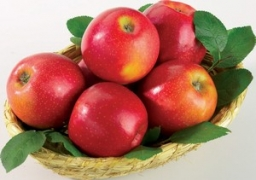Did you find an error or inaccuracy? Feel free to write us. Thank you!Math student
solution not clear

3 years ago  2 LikesDr Math
it's simple fraction equation; type is n=n where on left n is written as n/5 + n/4 + 33

3 years ago  2 LikesTips to related online calculators
Need help to calculate sum, simplify or multiply fractions? Try our fraction calculator.
Do you have a linear equation or system of equations and looking for its solution? Or do you have a quadratic equation?

## Related math problems and questions:

• Fruits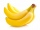For the price of one pineapple, I will buy two oranges. For the price of three oranges, I will buy four apples. I will buy 6 bananas for the price of three apples. What is the price of one banana if I pay 1 euro for one pineapple?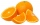If there are 7 apples and 5 oranges in the basket then what fraction of oranges are there in the fruit basket?
• Chocolate to sweetsI have 30 basket sweets and 15 chocolate in a basket. If take 5 sweet out of the basket what is the ratio in simplest form of the chocolate to the numbers of sweet left in the basket?
• Apples 14Maya bought 18 apples. She used 50% of the apples to make apple pies and 1/3 of the apples was mixed with other fruits to make salad. How many apples were left?
• ApplesSchool kitchen bought 36kg apples for 12 kc/kg. How many kilograms of apples 1/4 cheaper can they buy for the same money?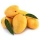A basket is full of 50 different kinds of fruits that has a ratio of 2:3:5. If the fruits are apple, mango, and guava, how many guavas are there?
• Fraction + eqSolve following simple equation with fractions: -5/6(8+5b) = 75 + 5/3b
• Guess a fractionTom was asked to guess a fraction. The sum of 1/2 the numerator and 1/3 of its denominator is 30. If Tom subtracts 36 from its denominator, the fraction becomes 1/3. What is the fraction that Tom was asked to guess? (Leave your answer in simplest form)
• Apples investment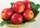The merchant bought a certain amount of apples and paid 1 denarius for every 7 apples. The next day he sold all the apples for 1 denarius for 5 pieces and earned 12 denarii. How many apples did he originally buy and how many did he have to invest in this
• A man 3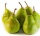A man buys a box of fruits containing 286 fruits out of these 1/2 are apples and the rest are pears. 4/13 of the pears are rotten. He sells the good pears at rupees 4 1/11 each. How much money does he receive on selling the good pears?In six baskets, the seller has fruit. In individual baskets, there are only apples or just pears with the following number of fruits: 5,6,12,14,23 and 29. "If I sell this basket," the salesman thinks, "then I will have just as many apples as a pear." WhicThe fruit salad is prepared from oranges and bananas. One kilogram of oranges costs 30 CZK, and one kilogram of bananas costs 18 CZK. How many kilograms of bananas need to be added to half a kilogram of oranges to produce a fruit salad worth CZK 21 per 1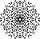What fraction of number 1 to 30 is prime?The weight of the empty fruit basket is ¾ kg. We added ½ kg apples and 5/4 kg guavas. What will be the total weight of the fruit basket?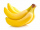Ashok Rao planted bananas on 2/7 th of his field on his 21 acers. What is the area of the bananas plantation?There were pears in the basket, I took two-fifths of them, and left six in the basket. How many pears did I take?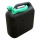The bucket has 5/8 gallons of water. The bucket tips over, and 7/12 gallon of water pours out. How much water is left in the bucket, in gallons, written as a fraction?